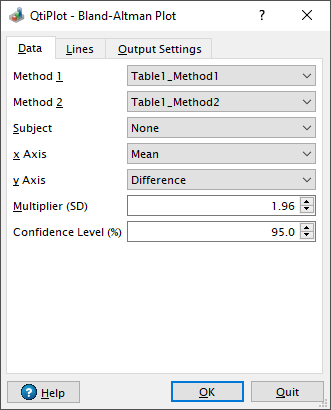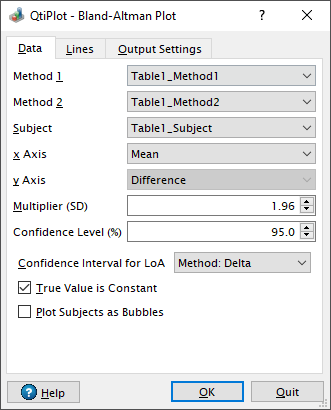# Bland-Altman Plot Dialog

This dialog is activated by selecting the Bland-Altman Plot... command from the Plot -> Statistical Graphs menu. It can be used in order to create and customize a Bland-Altman plot of the selected data columns in the active table window.

## Data Tab

### Design 1: One data-pair per subject

A Bland-Altman plot is used to compare two measurements of the same variable. That is, it is a method comparison technique. In the initial design of this analysis, that has been used for many years, each of the two measurement methods is used once on each subject. You need to provide two input data columns, one for each measurement method, via the Method 1 and Method 2 list boxes. The Subject box should be set to None in this design. A big drawback of this simple design is that no repeatability parameter can be computed.

Figure 5-99. Bland-Altman Plot... dialog: Data tab for single measurements.Considering n paired observations for method 1 (M1k) and method 2 (M2k), with k = 1, 2,...,n, the simplest form of a Bland-Altman plot is obtained by plotting the differences dk = M1k - M2k on the Y axis versus the pair means (M1k + M2k)/2 on the X axis.

A horizontal line representing the bias is drawn at the mean difference: dmean = ∑dk/n.

Two other horizontal lines, known as the limits of agreement (LoA ) are added to the plot at:

LoAup,low = dmean Ý 1.96 Sd

where Sd is the standard deviation:

Sd = [1/(n-1)∑(dk - dmean )2]1/2

Sometimes the 1.96 multiplier for the standard deviation is replaced with 2 or with another value. The default value in the Multiplier (SD) box is therefore set to 1.96 and can be freely modified.

#### Confidence Intervals

The α% confidence interval for the mean difference (the bias) is calculated as dmean Ý t(1 + α)/2,n-1V1/2 , with the variance: V = Sd2/n and t(1 + α)/2,n-1 being the Student's t distribution with n - 1 degrees of freedom. The default value for α is set to 95 in the Confidence Level (%) box.

The α% confidence interval for the limits of agreement is calculated as:

LoAup,low Ý t(1 + α)/2,n-1VLoA1/2

with the variance:

VLoA = [1/n + 0.5*1.962/(n-1)]Sd2

There are four display modes available for the X axis of a Bland-Altman plot:

Mean

The abscissas are calculated as the arithmetic mean of the paired values. This is the default mode.

Method1

The abscissas are the values measured using the first method.

Method2

The abscissas are the values measured using the second method.

GeometricMean

The abscissas are calculated as the geometric mean of the paired values.

There are three display modes available for the Y axis of a Bland-Altman plot:

DifferencePercent

The ordinates are calculated as the difference of the paired values, divided by their arithmetic mean and multiplied by 100.

Difference

The ordinates are calculated as the difference of the paired values. This is the default mode.

Ratio

The ordinates are calculated as the ratios of the paired values.

### Design 2: Repeated measurements for each method

Multiple observations by each method on each subject are needed in order to compare the repeatability of the two methods. For this more complex design of the Bland-Altman analysis three columns of input data are required: one with the measurements of the first method, one with the measurements of the second method and one with the subject values.

In this design only one display mode is available for the Y axis of a Bland-Altman plot: the default Difference mode. Also, the result curve displays the data points corresponding to each subject with a different color.

Figure 5-100. Bland-Altman Plot... dialog: Data tab with Subject column for repeated measurements.Two methods are available for calculating the Confidence interval for the limits of agreement (LoA) in the case of replicated measurements: Delta method and MOVER (Method of Variance Estimates Recovery). The calculation steps for each of these two methods are explained in detail by GY Zou in his paper Confidence interval estimation for the Bland-Altman limits of agreement with multiple observations per individual, Statistical Methods in Medical Research, 2013 Dec;22(6):630-42.

The use of the option True Value is Constant is very clearly explained by Bland and Altman in their paper from 2007 Agreement between methods of measurement with multiple observations per individual, Journal of Biopharmaceutical Statistics, 17: 571-582: "There are two different situations to consider for replicated data. We can think of the observations for the same subject as a series of measurements of a quantity that does not vary over the period of observation... Or we can think of them as pairs of measurements by two methods of a changing quantity, where it is the instantaneous measurement for the subject which we want to capture....The distinction is important, as it determines whether we need to consider pairing of observations by the two methods. Indeed, for the first (constant) case we do not require equal replication of each method for each individual, whereas this is a requirement for the second (non-constant) case."

If the option True Value is Constant is checked, the data columns for Method 1 and Method 2 may have a different number of input cells, since pairing is not required. The calculations for the limits of agreement and the corresponding confidence intervals will take into account the different number of observations for each method, nevertheless, only paired observations will be displayed by the output result curve.

By checking the option Plot Subjects as Bubbles, it is possible to group the measurements for each subject and represent them as one bubble with the center located at the following coordinates: mean of group (X) and mean difference (Y). The sizes of the bubbles are proportional to the number of replicates. This option does not affect in any way the calculations of the various reported values, only the drawing of the result data curve.

## Lines Tab

This tab helps controlling the drawing of the various horizontal lines: the equality line (Zero difference), the bias (Mean difference) and the limits of agreement. The drawing of the confidence intervals, their color filling and opacity can also be customized via this tab.

Figure 5-101. Bland-Altman Plot... dialog: Lines tab.## Output Settings Tab

The Table button, when pressed, activates the results table. The user may type the name of the result table in the edit box. Please beware that if the name corresponds to an existing table, the contents of that table will be altered.

Figure 5-102. Bland-Altman Plot... dialog: Output Settings tab.The last two columns in the result table, Statistics and Values, display the names and, respectively, the values calculated for the bias (Mean), the limits of agreement (Mean Ý Multiplier*SD) and their corresponding confidence intervals. Other two calculated values are the Mean of Pairwise Means or MPM, which is the mean of all paired values and the Bland-Altman Ratio, which is calculated as: (LoAup - LoAlow)/MPM. In the case of repeated observations, these columns also display the information about the True Value is Constant setting (1 if checked, 0 if not) and about the method used to calculate the confidence intervals for the limits of agreement (Delta or Mover).